Pairs Trading using Data-Driven Techniques: Simple Trading Strategies Part 3

Pairs trading is a nice example of a strategy based on mathematical analysis. We’ll demonstrate how to leverage data to create and automate a pairs trading strategy.

Underlying Principle

Let’s say you have a pair of securities X and Y that have some underlying economic link, for example two companies that manufacture the same product like Pepsi and Coca Cola. You expect the ratio or difference in prices (also called the spread) of these two to remain constant with time. However, from time to time, there might be a divergence in the spread between these two pairs caused by temporary supply/demand changes, large buy/sell orders for one security, reaction for important news about one of the companies etc. In this scenario, one stock moves up while the other moves down relative to each other. If you expect this divergence to revert back to normal with time, you can make a pairs trade.

When there is a temporary divergence, the pairs trade would be to sell the outperforming stock (the stock that moved up )and to buy the underperforming stock (the stock that moved down ). You are making a bet that the spread between the two stocks would eventually converge by either the outperforming stock moving back down or the underperforming stock moving back up or both — your trade will make money in all of these scenarios. If both the stocks move up or move down together without changing the spread between them, you don’t make or lose any money.

Hence, pairs trading is a market neutral trading strategy enabling traders to profit from virtually any market conditions: uptrend, downtrend, or sideways movement.

Explaining the Concept: We start by generating two fake securities.

import numpy as np
import pandas as pd

import statsmodels
from statsmodels.tsa.stattools import coint
# just set the seed for the random number generator
np.random.seed(107)

import matplotlib.pyplot as plt

Let’s generate a fake security X and model it’s daily returns by drawing from a normal distribution. Then we perform a cumulative sum to get the value of X on each day.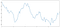Fake Security X with returns drawn from a normal distribution
# Generate daily returnsXreturns = np.random.normal(0, 1, 100) # sum them and shift all the prices upX = pd.Series(np.cumsum(
Xreturns), name='X')
+ 50
X.plot(figsize=(15,7))
plt.show()

Now we generate Y which has a deep economic link to X, so price of Y should vary pretty similarly as X. We model this by taking X, shifting it up and adding some random noise drawn from a normal distribution.

noise = np.random.normal(0, 1, 100)
Y = X + 5 + noise
Y.name = 'Y'
pd.concat([X, Y], axis=1).plot(figsize=(15,7))plt.show()

Cointegration

Cointegration, very similar to correlation, means that the ratio between two series will vary around a mean. The two series, Y and X follow the follwing:

Y = ⍺ X + e

where ⍺ is the constant ratio and e is white noise. Read more here

For pairs trading to work between two timeseries, the expected value of the ratio over time must converge to the mean, i.e. they should be cointegrated.

The time series we constructed above are cointegrated. We’ll plot the ratio between the two now so we can see how this looks.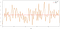Ratio between prices of two cointegrated stocks and it’s mean
(Y/X).plot(figsize=(15,7)) plt.axhline((Y/X).mean(), color='red', linestyle='--') plt.xlabel('Time')
plt.legend(['Price Ratio', 'Mean'])
plt.show()

Testing for Cointegration

There is a convenient test that lives in statsmodels.tsa.stattools. We should see a very low p-value, as we've artificially created two series that are as cointegrated as physically possible.

# compute the p-value of the cointegration test
# will inform us as to whether the ratio between the 2 timeseries is stationary
# around its mean
score, pvalue, _ = coint(X,Y)
print pvalue

1.81864477307e-17

Note: Correlation vs. Cointegration

Correlation and cointegration, while theoretically similar, are not the same. Let’s look at examples of series that are correlated, but not cointegrated, and vice versa. First let's check the correlation of the series we just generated.

X.corr(Y)

0.951

That’s very high, as we would expect. But how would two series that are correlated but not cointegrated look? A simple example is two series that just diverge.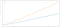Two correlated series (that are not co-integrated)
ret1 = np.random.normal(1, 1, 100)
ret2 = np.random.normal(2, 1, 100)

s1 = pd.Series( np.cumsum(ret1), name='X')
s2 = pd.Series( np.cumsum(ret2), name='Y')

pd.concat([s1, s2], axis=1 ).plot(figsize=(15,7))
plt.show()
print 'Correlation: ' + str(X_diverging.corr(Y_diverging))
score, pvalue, _ = coint(X_diverging,Y_diverging)
print 'Cointegration test p-value: ' + str(pvalue)

Correlation: 0.998
Cointegration test p-value: 0.258

A simple example of cointegration without correlation is a normally distributed series and a square wave.

Y2 = pd.Series(np.random.normal(0, 1, 800), name='Y2') + 20
Y3 = Y2.copy()
Y3[0:100] = 30
Y3[100:200] = 10
Y3[200:300] = 30
Y3[300:400] = 10
Y3[400:500] = 30
Y3[500:600] = 10
Y3[600:700] = 30
Y3[700:800] = 10
Y2.plot(figsize=(15,7))
Y3.plot()
plt.ylim([0, 40])
plt.show()
# correlation is nearly zero
print 'Correlation: ' + str(Y2.corr(Y3))
score, pvalue, _ = coint(Y2,Y3)
print 'Cointegration test p-value: ' + str(pvalue)

Correlation: 0.007546
Cointegration test p-value: 0.0

The correlation is incredibly low, but the p-value shows perfect cointegration!

How to make a pairs trade?

Because two cointegrated time series (such as X and Y above) drift towards and apart from each other, there will be times when the spread is high and times when the spread is low. We make a pairs trade by buying one security and selling another. This way, if both securities go down together or go up together, we neither make nor lose money — we are market neutral.

Going back to X and Y above that follow Y = ⍺ X + e, such that ratio (Y/X) moves around it’s mean value ⍺, we make money on the ratio of the two reverting to the mean. In order to do this we’ll watch for when X and Y are far apart, i.e ⍺ is too high or too low:

• Going Long the Ratio This is when the ratio is smaller than usual and we expect it to increase. In the above example, we place a bet on this by buying Y and selling X.
• Going Short the Ratio This is when the ratio is large and we expect it to become smaller. In the above example, we place a bet on this by selling Y and buying X.

Note that we always have a “hedged position”: a short position makes money if the security sold loses value, and a long position will make money if a security gains value, so we’re immune to overall market movement. We only make or lose money if securities X and Y move relative to each other.

Using Data to find securities that behave like this

The best way to do this is to start with securities you suspect may be cointegrated and perform a statistical test. If you just run statistical tests over all pairs, you’ll fall prey to multiple comparison bias.

Multiple comparisons bias is simply the fact that there is an increased chance to incorrectly generate a significant p-value when many tests are run, because we are running a lot of tests. If 100 tests are run on random data, we should expect to see 5 p-values below 0.05. If you are comparing n securities for co-integration, you will perform n(n-1)/2 comparisons, and you should expect to see many incorrectly significant p-values, which will increase as you increase. To avoid this, pick a small number of pairs you have reason to suspect might be cointegrated and test each individually. This will result in less exposure to multiple comparisons bias.

So let’s try to find some securities that display cointegration. Let’s work with a basket of US large cap tech stocks — in S&P 500. These stocks operate in a similar segment and could have cointegrated prices. We scan through a list of securities and test for cointegration between all pairs. It returns a cointegration test score matrix, a p-value matrix, and any pairs for which the p-value was less than 0.05. This method is prone to multiple comparison bias and in practice the securities should be subject to a second verification step. Let’s ignore this for the sake of this example.

def find_cointegrated_pairs(data):
n = data.shape
score_matrix = np.zeros((n, n))
pvalue_matrix = np.ones((n, n))
keys = data.keys()
pairs = []
for i in range(n):
for j in range(i+1, n):
S1 = data[keys[i]]
S2 = data[keys[j]]
result = coint(S1, S2)
score = result
pvalue = result
score_matrix[i, j] = score
pvalue_matrix[i, j] = pvalue
if pvalue < 0.02:
pairs.append((keys[i], keys[j]))
return score_matrix, pvalue_matrix, pairs

Note: We include the market benchmark (SPX) in our data — the market drives the movement of so many securities that often you might find two seemingly cointegrated securities; but in reality they are not cointegrated with each other but both conintegrated with the market. This is known as a confounding variable and it is important to check for market involvement in any relationship you find.

from backtester.dataSource.yahoo_data_source import YahooStockDataSource
from datetime import datetime
startDateStr = '2007/12/01'
endDateStr = '2017/12/01'
cachedFolderName = 'yahooData/'
'HPQ','JNPR','AMD','IBM']
ds = YahooStockDataSource(cachedFolderName=cachedFolderName,
dataSetId=dataSetId,
instrumentIds=instrumentIds,
startDateStr=startDateStr,
endDateStr=endDateStr,
event='history')

Now let’s try to find cointegrated pairs using our method.

# Heatmap to show the p-values of the cointegration test
# between each pair of stocks
scores, pvalues, pairs = find_cointegrated_pairs(data)
import seaborn
m = [0,0.2,0.4,0.6,0.8,1]
seaborn.heatmap(pvalues, xticklabels=instrumentIds,
yticklabels=instrumentIds, cmap=’RdYlGn_r’,
mask = (pvalues >= 0.98))
plt.show()
print pairs

Looks like ‘ADBE’ and ‘MSFT’ are cointegrated. Let’s take a look at the prices to make sure this actually makes sense.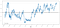Plot of Price Ratio between MSFT and ADBE from 2008–2017
S2 = data['MSFT']
score, pvalue, _ = coint(S1, S2)
print(pvalue)
ratios = S1 / S2
ratios.plot()
plt.axhline(ratios.mean())
plt.legend([' Ratio'])
plt.show()

The ratio does look like it moved around a stable mean.The absolute ratio isn’t very useful in statistical terms. It is more helpful to normalize our signal by treating it as a z-score. Z score is defined as:

Z Score (Value) = (Value — Mean) / Standard Deviation

WARNING

In practice this is usually done to try to give some scale to the data, but this assumes an underlying distribution. Usually normal. However, much financial data is not normally distributed, and we must be very careful not to simply assume normality, or any specific distribution when generating statistics. The true distribution of ratios could be very fat-tailed and prone to extreme values messing up our model and resulting in large losses.

def zscore(series):
return (series - series.mean()) / np.std(series)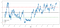Z Score of Price Ratio between MSFT and ADBE from 2008–2017
zscore(ratios).plot()
plt.axhline(zscore(ratios).mean())
plt.axhline(1.0, color=’red’)
plt.axhline(-1.0, color=’green’)
plt.show()

It’s easier to now observe the ratio now moves around the mean, but sometimes is prone to large divergences from the mean, which we can take advantages of.

Now that we’ve talked about the basics of pair trading strategy, and identified co-integrated securities based on historical price, let’s try to develop a trading signal. First, let’s recap the steps in developing a trading signal using data techniques:

• Collect reliable Data and clean Data
• Create features from data to identify a trading signal/logic
• Features can be moving averages or ratios of price data, correlations or more complex signals — combine these to create new features
• Generate a trading signal using these features, i.e which instruments are a buy, a sell or neutral

Step 1: Setup your problem

Here we are trying to create a signal that tells us if the ratio is a buy or a sell at the next instant in time, i.e our prediction variable Y:

Y = Ratio is buy (1) or sell (-1)

Y(t)= Sign( Ratio(t+1) — Ratio(t) )

Note we don’t need to predict actual stock prices, or even actual value of ratio (though we could), just the direction of next move in ratio

Step 2: Collect Reliable and Accurate Data

Auquan Toolbox is your friend here! You only have to specify the stock you want to trade and the datasource to use, and it pulls the required data and cleans it for dividends and stock splits. So our data here is already clean.

We are using the following data from Yahoo at daily intervals for trading days over last 10 years (~2500 data points): Open, Close, High, Low and Trading Volume

Step 3: Split Data

Don’t forget this super important step to test accuracy of your models. We’re using the following Training/Validation/Test Split

• Training 7 years ~ 70%
• Test ~ 3 years 30%
ratios = data['ADBE'] / data['MSFT']
print(len(ratios))
train = ratios[:1762]
test = ratios[1762:]

Ideally we should also make a validation set but we will skip this for now.

Step 4: Feature Engineering

What could relevant features be? We want to predict the direction of ratio move. We’ve seen that our two securities are cointegrated so the ratio tends to move around and revert back to the mean. It seems our features should be certain measures for the mean of the ratio, the divergence of the current value from the mean to be able to generate our trading signal.

Let’s use the following features:

• 60 day Moving Average of Ratio: Measure of rolling mean
• 5 day Moving Average of Ratio: Measure of current value of mean
• 60 day Standard Deviation
• z score: (5d MA — 60d MA) /60d SD
ratios_mavg5 = train.rolling(window=5,
center=False).mean()
ratios_mavg60 = train.rolling(window=60,
center=False).mean()
std_60 = train.rolling(window=60,
center=False).std()
zscore_60_5 = (ratios_mavg5 - ratios_mavg60)/std_60
plt.figure(figsize=(15,7))
plt.plot(train.index, train.values)
plt.plot(ratios_mavg5.index, ratios_mavg5.values)
plt.plot(ratios_mavg60.index, ratios_mavg60.values)
plt.legend(['Ratio','5d Ratio MA', '60d Ratio MA'])plt.ylabel('Ratio')
plt.show()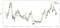60d and 5d MA of Price Ratios
plt.figure(figsize=(15,7))
zscore_60_5.plot()
plt.axhline(0, color='black')
plt.axhline(1.0, color='red', linestyle='--')
plt.axhline(-1.0, color='green', linestyle='--')
plt.legend(['Rolling Ratio z-Score', 'Mean', '+1', '-1'])
plt.show()

The Z Score of the rolling means really brings out the mean reverting nature of the ratio!

Step 5: Model Selection

Let’s start with a really simple model. Looking at the z-score chart, we can see that whenever the z-score feature gets too high, or too low, it tends to revert back. Let’s use +1/-1 as our thresholds for too high and too low, then we can use the following model to generate a trading signal:

• Ratio is buy (1) whenever the z-score is below -1.0 because we expect z score to go back up to 0, hence ratio to increase
• Ratio is sell(-1) when the z-score is above 1.0 because we expect z score to go back down to 0, hence ratio to decrease

Step 6: Train, Validate and Optimize

Finally, let’s see how our model actually does on real data? Let’s see what this signal looks like on actual ratios

# Plot the ratios and buy and sell signals from z score
plt.figure(figsize=(15,7))
train[60:].plot()
sell = train.copy()
sell[zscore_60_5<1] = 0
sell[60:].plot(color=’r’, linestyle=’None’, marker=’^’)
x1,x2,y1,y2 = plt.axis()
plt.axis((x1,x2,ratios.min(),ratios.max()))
plt.legend([‘Ratio’, ‘Buy Signal’, ‘Sell Signal’])
plt.show()

The signal seems reasonable, we seem to sell the ratio (red dots) when it is high or increasing and buy it back when it's low (green dots) and decreasing. What does that mean for actual stocks that we are trading? Let’s take a look

# Plot the prices and buy and sell signals from z score
plt.figure(figsize=(18,9))
S2 = data['MSFT'].iloc[:1762]
S1[60:].plot(color='b')
S2[60:].plot(color='c')
sellR = 0*S1.copy()
# When buying the ratio, buy S1 and sell S2
# When selling the ratio, sell S1 and buy S2
sellR[sell!=0] = S1[sell!=0]
sellR[60:].plot(color='r', linestyle='None', marker='^')
x1,x2,y1,y2 = plt.axis()
plt.axis((x1,x2,min(S1.min(),S2.min()),max(S1.max(),S2.max())))
plt.show()

Notice how we sometimes make money on the short leg and sometimes on the long leg, and sometimes both.

We’re happy with our signal on the training data. Let’s see what kind of profits this signal can generate. We can make a simple backtester which buys 1 ratio (buy 1 ADBE stock and sell ratio x MSFT stock) when ratio is low, sell 1 ratio (sell 1 ADBE stock and buy ratio x MSFT stock) when it’s high and calculate PnL of these trades.

# Trade using a simple strategy
def trade(S1, S2, window1, window2):

# If window length is 0, algorithm doesn't make sense, so exit
if (window1 == 0) or (window2 == 0):
return 0

# Compute rolling mean and rolling standard deviation
ratios = S1/S2
ma1 = ratios.rolling(window=window1,
center=False).mean()
ma2 = ratios.rolling(window=window2,
center=False).mean()
std = ratios.rolling(window=window2,
center=False).std()
zscore = (ma1 - ma2)/std

# Start with no money and no positions
money = 0
countS1 = 0
countS2 = 0
for i in range(len(ratios)):
# Sell short if the z-score is > 1
if zscore[i] > 1:
money += S1[i] - S2[i] * ratios[i]
countS1 -= 1
countS2 += ratios[i]
print('Selling Ratio %s %s %s %s'%(money, ratios[i], countS1,countS2))
# Buy long if the z-score is < 1
elif zscore[i] < -1:
money -= S1[i] - S2[i] * ratios[i]
countS1 += 1
countS2 -= ratios[i]
print('Buying Ratio %s %s %s %s'%(money,ratios[i], countS1,countS2))
# Clear positions if the z-score between -.5 and .5
elif abs(zscore[i]) < 0.75:
money += S1[i] * countS1 + S2[i] * countS2
countS1 = 0
countS2 = 0
print('Exit pos %s %s %s %s'%(money,ratios[i], countS1,countS2))

return money

1783.375

So that strategy seems profitable! Now we can optimize further by changing our moving average windows, by changing the thresholds for buy/sell and exit positions etc and check for performance improvements on validation data.

We could also try more sophisticated models like Logisitic Regression, SVM etc to make our 1/-1 predictions.

For now, let’s say we decide to go forward with this model, this brings us to

Step 7: Backtest on Test Data

Backtesting is simple, we can just use our function from above to see PnL on test data

5262.868

The model does quite well! This makes our first simple pairs trading model.

Avoid Overfitting

Before ending the discussion, we’d like to give special mention to overfitting. Overfitting is the most dangerous pitfall of a trading strategy. An overfit algorithm may perform wonderfully on a backtest but fails miserably on new unseen data — this mean it has not really uncovered any trend in data and no real predictive power. Let’s take a simple example.

In our model, we used rolling parameter estimates and may wish to optimize window length. We may decide to simply iterate over all possible, reasonable window length and pick the length based on which our model performs the best . Below we write a simple loop to to score window lengths based on pnl of training data and find the best one.

# Find the window length 0-254
# that gives the highest returns using this strategy
data['MSFT'].iloc[:1762], l, 5)
for l in range(255)]
best_length = np.argmax(length_scores)
print ('Best window length:', best_length)

('Best window length:', 40)

Now we check the performance of our model on test data and we find that this window length is far from optimal! This is because our original choice was clearly overfitted to the sample data.

# Find the returns for test data
# using what we think is the best window length
data['MSFT'].iloc[1762:],l,5)
for l in range(255)]
print (best_length, 'day window:', length_scores2[best_length])
# Find the best window length based on this dataset,
# and the returns using this window length
best_length2 = np.argmax(length_scores2)
print (best_length2, 'day window:', length_scores2[best_length2])

(40, 'day window:', 1252233.1395)
(15, 'day window:', 1449116.4522)

Clearly fitting to our sample data doesn't always give good results in the future. Just for fun, let's plot the length scores computed from the two datasets

plt.figure(figsize=(15,7))
plt.plot(length_scores)
plt.plot(length_scores2)
plt.xlabel('Window length')
plt.ylabel('Score')
plt.legend(['Training', 'Test'])
plt.show()

We can see that anything between 20–50 would be a good choice for window.

To avoid overfitting, we can use economic reasoning or the nature of our algorithm to pick our window length. We can also use Kalman filters, which do not require us to specify a length; this method will be covered in another notebook later.

Next Steps

In this post, we presented some simple introductory approaches to demonstrate the process of developing a pairs trading strategy. In practice one should use more sophisticated statistics, some of which are listed here

• Hurst exponent
• Half-life of mean reversion inferred from an Ornstein–Uhlenbeck process
• Kalman filters

Written by

Shub Jain

Auquan aims to to engage people from diverse backgrounds to apply the skills from their respective fields to develop high quality trading strategies. We believe that extremely talented people equipped with right knowledge and attitude can design successful trading algorithms.

Welcome to a place where words matter. On Medium, smart voices and original ideas take center stage - with no ads in sight. Watch
Follow all the topics you care about, and we’ll deliver the best stories for you to your homepage and inbox. Explore
Get unlimited access to the best stories on Medium — and support writers while you’re at it. Just \$5/month. Upgrade